# Texas Go Math Grade 1 Lesson 10.1 Answer Key Count Forward by Ones to 120

Refer to our Texas Go Math Grade 1 Answer Key Pdf to score good marks in the exams. Test yourself by practicing the problems from Texas Go Math Grade 1 Lesson 10.1 Answer Key Count Forward by Ones to 120.

## Texas Go Math Grade 1 Lesson 10.1 Answer Key Count Forward by Ones to 120

Explore

Write the numbers as you count forward.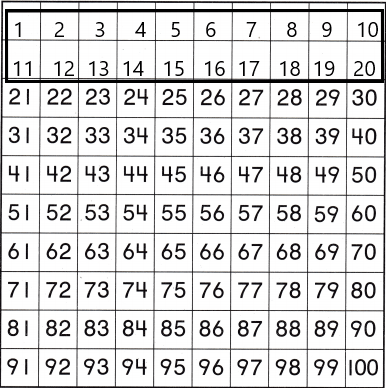FOR THE TEACHER • Read the following problem. Debbie saw this page in a puzzle book. Two rows of numbers are incomplete. Use what you know about counting forward to write the numbers.
Counting On – We can count on by saying by numbers while touching on each object once. Here, for instance, we can count the buttons by touching each button once. Counting on also requires us to count forward. Forward counting is counting by adding one more, every time.Math Talk
Mathematical Processes

Explain how you know which numbers to write.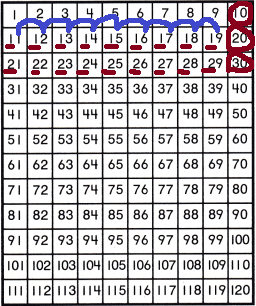Let’s take a look at the counting tracks. Notice the first row having 1 to 10. The second row has 11 to 20. Each number begin with 1, the second digit increases by 1 until the last column. In the third row, the pattern is the same only difference is the first digit is 2 instead of 1 and the second digit is increased by 1 until the last column. Notice that the second digit in each column is the same. Let’s skip down to 100 and the pattern continues. Now we have three-digit numbers like 101, 102, 103, 104, 105 and so on… and every third digit is increased by 1.

Model and Draw

Count forward. Write the numbers.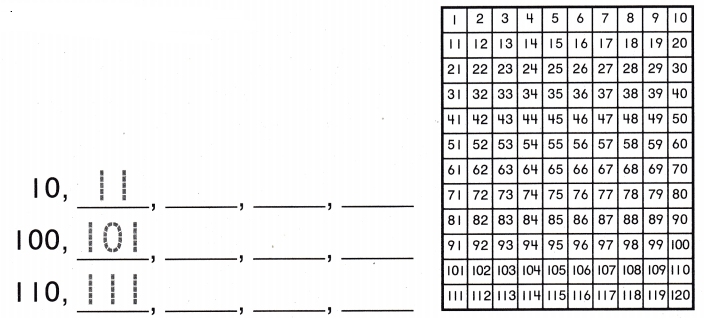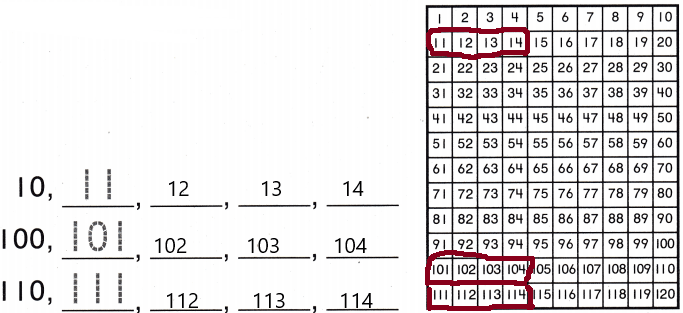Explanation:
Each number begin with 1, the second digit increases by 1 until the last column.
Share and Show

Use a Counting Chart. Count forward. Write the numbers.

Question 1.
114, ________, ________, ________, ________, ________, ________Explanation:
Starting from 114 and count increases by 1 means 115, 116, 117, 118, 119, 120. In the last column of the counting track, there are three digits the first two- digits are the same and the third digit is increased by 1.

Question 2.
51, ________, ________, ________, ________, ________, ________
51, 52, 53,54, 55, 56, 57.
Explanation: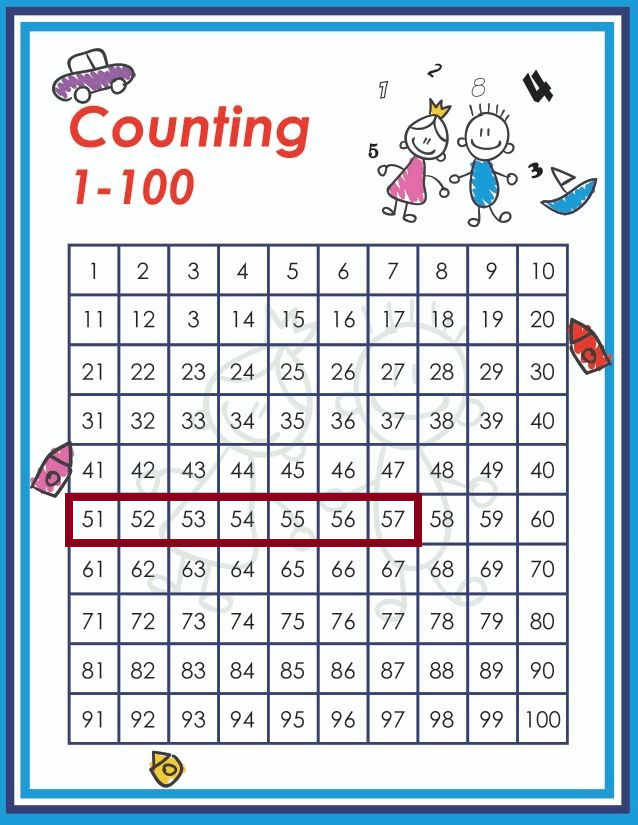The first digit is same and the second digit is increased by 1 in the counting track.

Question 3.
94, ________, ________, ________, ________, ________, ________
94, 95, 96, 97, 98, 99, 100
Explanation:
The numbers will be represented in the below-counting track.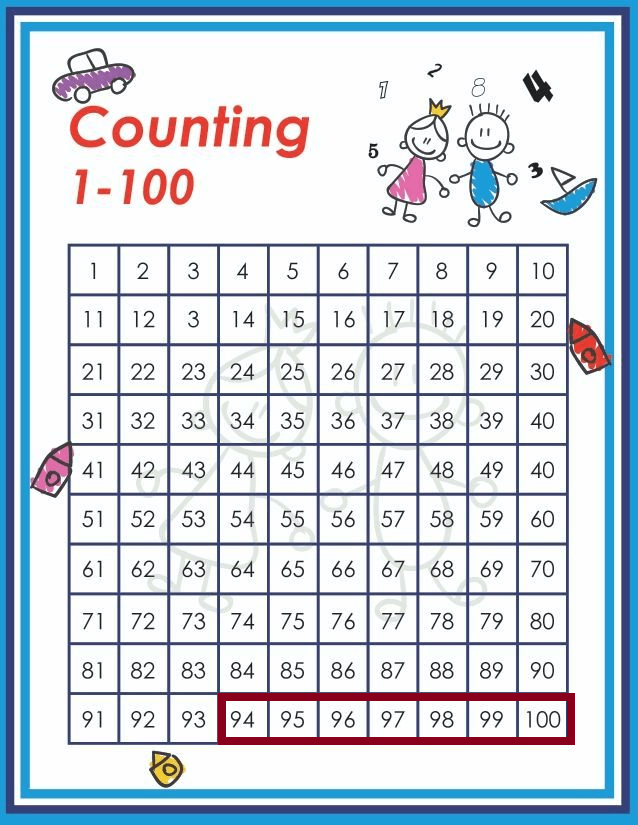Question 4.
78, ________, ________, ________, ________, ________, ________
78, 79, 80, 81, 82, 83, 84.
Explanation:
Counting forward means we need to add one.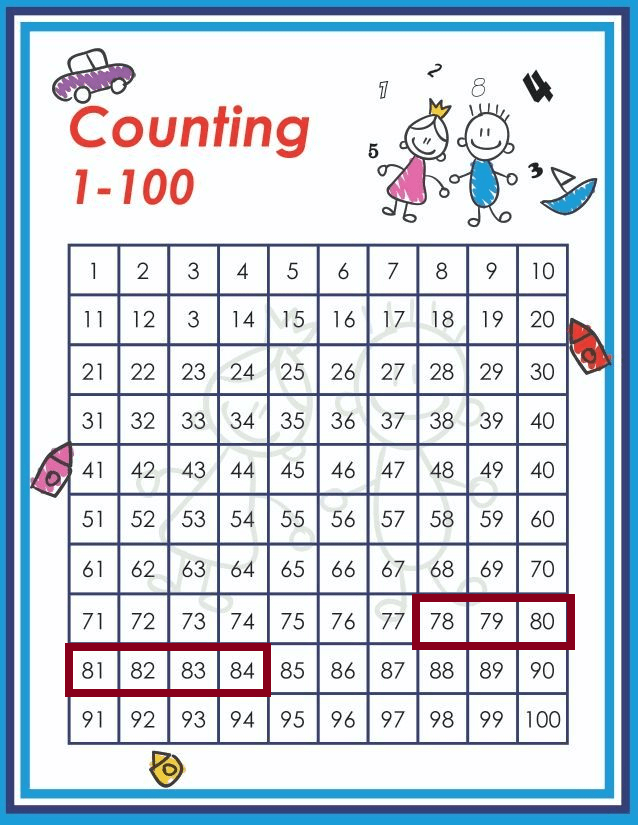Question 5.
35, ________, ________, ________, ________, ________, ________
35, 36, 37, 38, 39, 40, 41.
Explanation: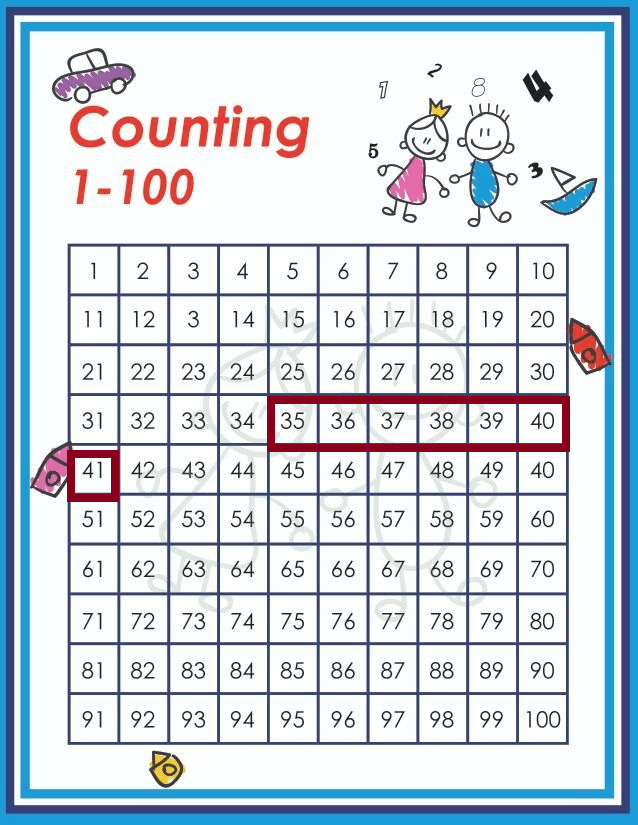counting forward means we are counting by 1 here.
counting by 1- 1, 2, 3, 4, 5…
here 35 is given so the next number is 36, 37, 38…..

Question 6.
104, ________, ________, ________, ________, ________, ________
104, 105, 106, 107, 108, 109, 110.
Explanation:Problem Solving

Use a Counting Chart. Count forward. Write the numbers.

Question 7.
19, _______, _______, _______, _______, _______, _______, _______, _______
19, 20, 21, 22, 23, 24, 25, 26, 27.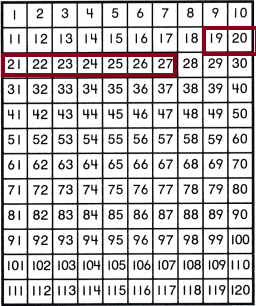Forward counting is counting by adding one more, every time.

Question 8.
98, _______, _______, _______, _______, _______, _______, _______, _______
98, 99, 100, 101, 102, 103, 104, 105, 106.Question 9.
107, _______, _______, _______, _______, _______, _______, _______, _______
107, 108, 109, 110, 111, 112, 113, 114, 115.
Increases by 1.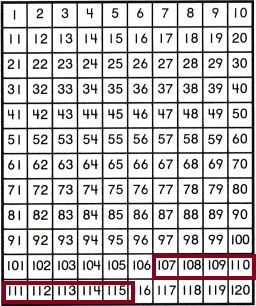Question 10.
43, _______, _______, _______, _______, _______, _______, _______, _______
43, 44, 45, 46, 47, 48, 49, 50, 51.
Increases by 1.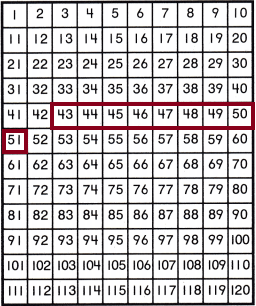Question 11.
68, _______, _______, _______, _______, _______, _______, _______, _______
68, 69, 70, 71, 72, 73, 74, 75, 76.
Increases by 1.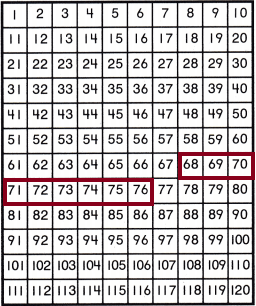Question 12.
50, _______, _______, _______, _______, _______, _______, _______, _______
50, 51, 52, 53, 54, 55, 56, 57, 58.
Increases by 1.Question 13.
H.O.T. Use a Counting Chart to write the numbers counting forward.
_______, _______, _______, _______, _______, 120115, 116, 117, 118, 119, 120
Counting forward means increases by 1.Question 14.
H.O.T. Multi-Step There is an unknown number in the sequence counting forward. The number is greater than 51. The number is less than 53. What is the unknown number?
Explanation:
Given: The unknown number in the counting track which is 51 is greater and less than 53. The unknown number is represented in the below diagram.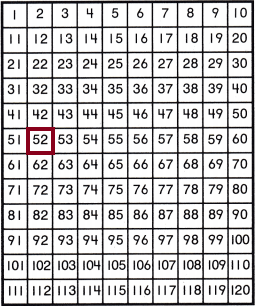Because 52 is greater than 51 and less than 53. So, 52 is the answer.

Question 15.
Count forward. Which numbers come next?
115, 116, _______, ______, _______, ______
(A) 17, 18, 19, 20
(B) 117, 118, 119, 120
(C) 7, 8, 9, 10Explanation: increases by 1 so the numbers sequence be 115, 116, 117, 118, 119, 120.

Question 16.
A jar has 97 marbles in it. There are 3 marbles on the table. Count forward as you put each marble in the jar. How many marbles are in the jar?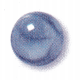(A) 100
(B) 99
(C) 93Explanation:=100 marbles
The number of marbles is present in jar=97.
The number of marbles is present on the table=3
In the question asked was count forward to arrange all the marbles in the jar. So it increasing by one- 97, 98, 99, 100.
97+3=100.

Question 17.
Display Some of the numbers are not shown. Count forward. Write the numbers that are not shown.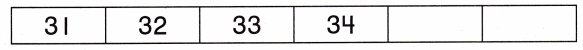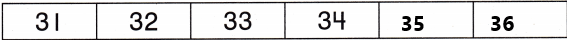Increase by 1. so 31, 32, 33, 34, 35, 36.

Question 18.
Texas Test Prep Count forward. What number is not shown?
107, 108, 109, ______, 111
(A) 100
(B) 110
(C) 101Explanation:
107, 108, 109, 110, 111.
The missing number is 110.
TAKE HOME ACTIVITY • Take a walk with your child. Count aloud together as you take 120 steps.
Take them for a walk and make them count the steps up to 120. so, they would get a count forward numbers.

### Texas Go Math Grade 1 Lesson 10.1 Homework and Practice Answer Key

Use a Counting Chart. Count forward. Write the numbers.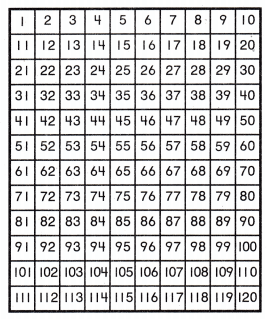Question 1.
62, _______, ______, ______,
62, 63, 64, 65.
Explanation:
Counting forward from 62. So it increases by 1 every time.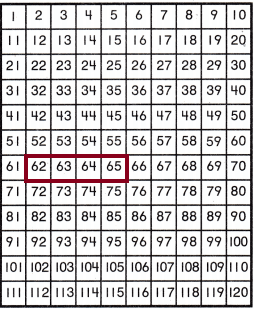Question 2.
21, _______, _______, _______
21, 22, 23, 24.
Explanation:
Counting forward starts from 21. So the number increases by 1 every time.Question 3.
104, _______, _______, _______, _______, _______
105, 106, 107, 108, 109.
The counting forward starts from 104. So, the number increases by 1 every time.Question 4.
48, _______, _______, _______, _______, _______, _______
48, 49, 50, 51, 52, 53, 54.
The counting forward starts from 48. So the number increases by 1 every time.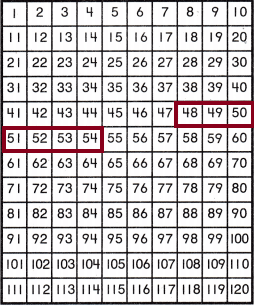Use a Counting Chart.

Question 5.
Jill has a set of number cards. Some of the cards are missing. Count forward. Fill in the numbers of the missing cards.
29, _______, _______, 32, _______, 34
29, 30, 31, 32, 33, 34.
The counting forward starts from 29. So the number increases by 1 every time.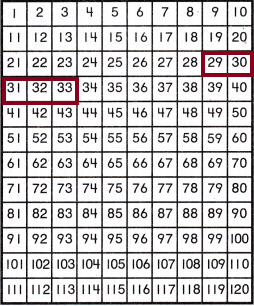Lesson Check

Question 6.
Count forward. Which numbers come next?
98, 99, _______, _______, _______, _______
(A) 94, 95, 96, 97
(B) 0, 1, 2, 3
(C) 100, 101, 102, 103Explanation:
The counting forward starts from 98. So the number increases by 1 every time.
The numbers are 98, 99, 100, 101, 102, 103.

Question 7.
Sally counted the shells in her collection. Count forward. Which numbers are not shown?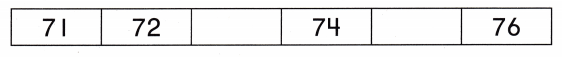(A) 72 and 74
(B) 73 and 75
(C) 72 and 76Count forward increases by 1.

Question 8.
Multi-Step There are 58 books in the bookcase. There are 2 books on the table and 2 on the chair. Count forward as you put each book in the bookcase. How many books are in the bookcase?(A) 62
(B) 54
(C) 60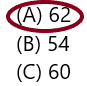Explanation:
Number of books in bookcase=58
The number of books on the table=2
The number of books in chair=2
To put all the books in the bookcase use the count forward and then arrange. The count forward starts from 58 so, Increase by 1 every time.
58, 59, 60, 61, 62.
Therefore, the total number of books in the bookcase is 62.

Question 9.
Count forward. What number is not shown?
97, 98, 99, ______, 101
(A) 90
(B) 100
(C) 101